Culvert Design Problem 1 - Determine culvert pipe size for discharge conditions:

Size a concrete culvert pipe crossing under a county road for the following design conditions: Q = 35 cfs; maximum headwater elevation is 104.6; upstream flowline elevation is 100.1; downstream flowline elevation is 99.7; culvert length is 58 ft and the culvert will project from fill with no headwalls.
1.
The problem states that the maximum headwater elevation is 104.6' and the upstream flowline elevation is 100.1. Thus the maximum headwater depth is 104.6 - 100.1 = 4.5'. Now, from the culverts submenu open the round trial design culvert selection calculation, CulvertSelRnd.aspx. Input the "Title" of the calculation, the "Designer/Checker" information and the variables.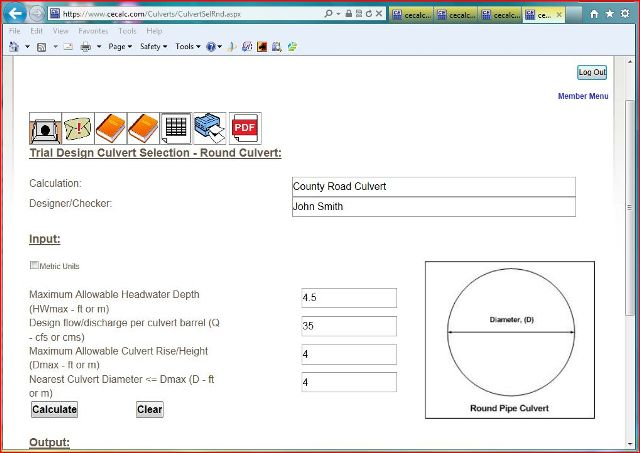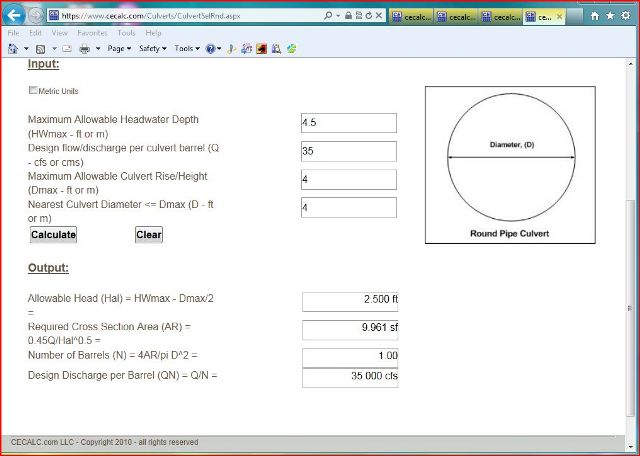2.
From the culverts submenu open up the inlet control culvert submerged inlet calculation, CulverICS.aspx. Input the "Title" of the calculation, the "Designer/Checker" information and the variables.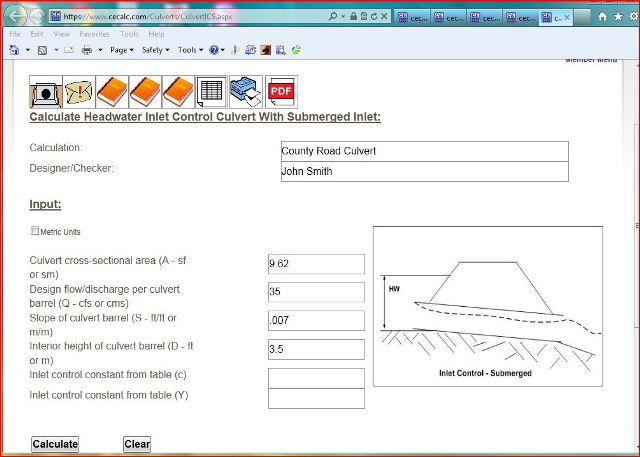3.
Open up the reference tables and find the inlet control constants "c" and "Y" from the table.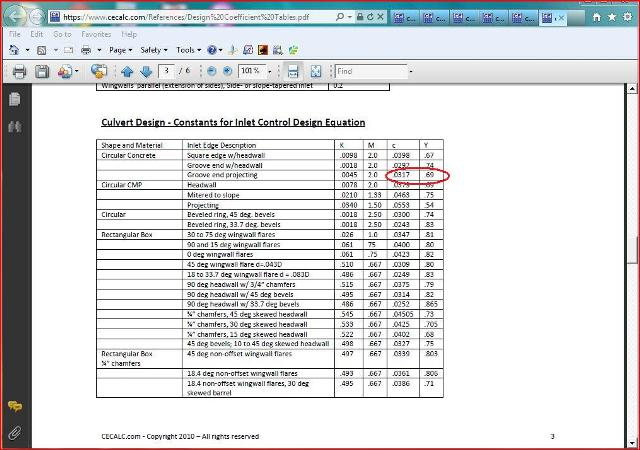4.
Input the remaining variable and click on the "Calculate" button. Read the results. The inlet control headwater depth is 2.835 ft.5.
Next open up the outlet control round culvert calculation, CulvertRndOC.aspx. Input the "Title" of the calculation, the "Designer/Checker" information and the variables.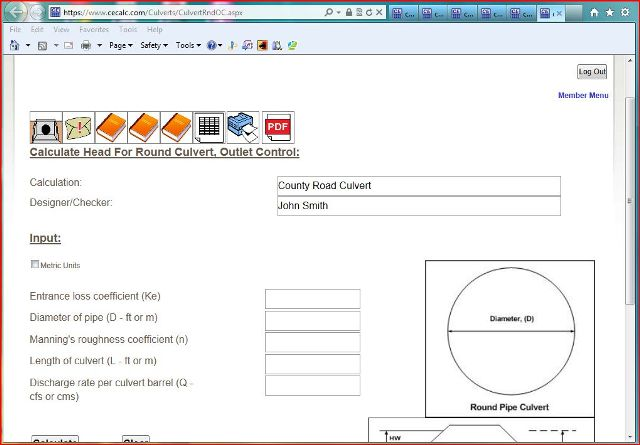6.
Open up the reference tables and find the entrance loss conefficient, "Ke" and the value for Manning's roughness coefficient "n".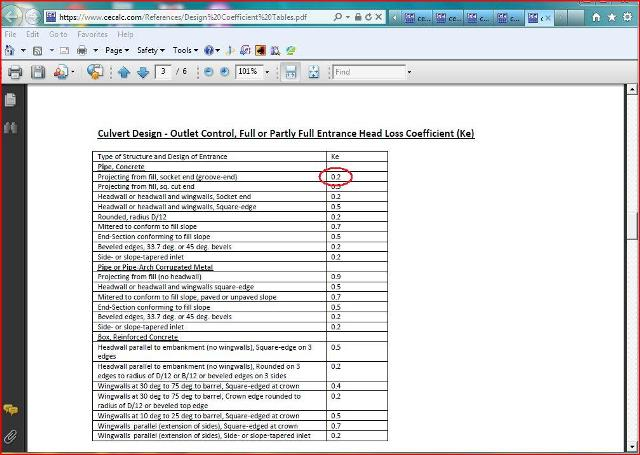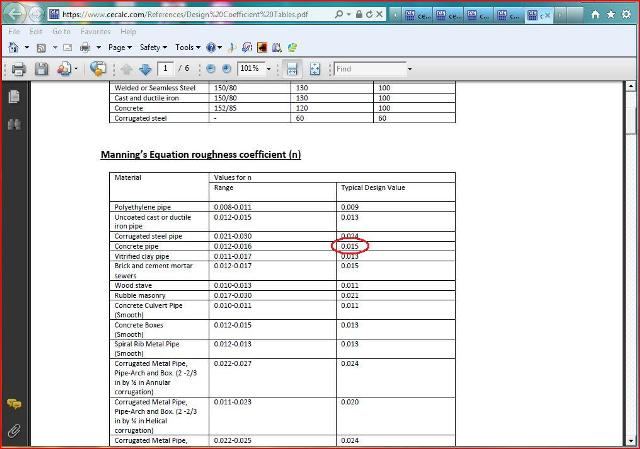7.
Enter the remaining variables and click on the "Calculate" button. Read the results. The outlet control culvert head is 0.248 ft.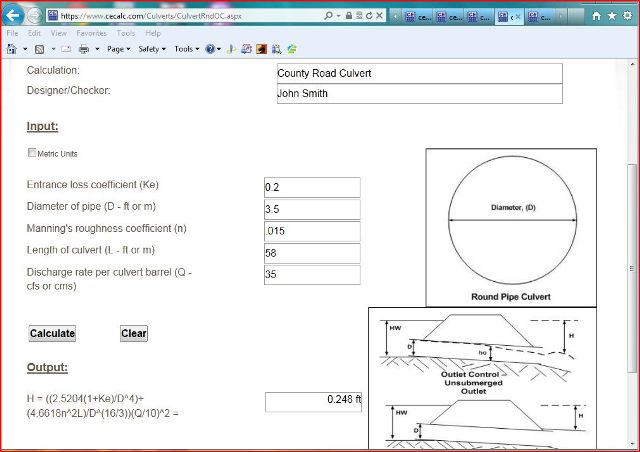8.
From the culverts submenu open up the tailwater depth calculation, Culvertho.aspx. Input the "Title" of the calculation, the "Designer/Checker" information and the variables.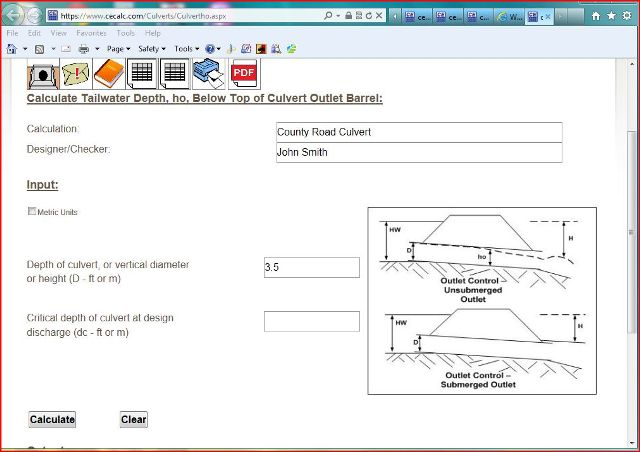9.
Open up the critical depth charts and determine the critical depth of a round 3.5' culvert flowing 35 cfs.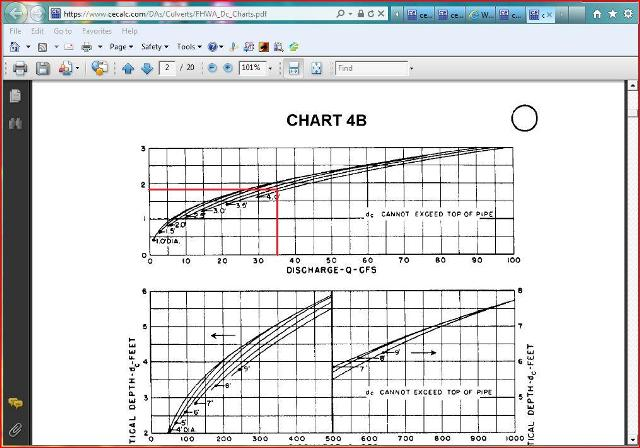10.
Enter the critical depth and click on the "Calculate" button. Read the results. The tailwater depth, ho is 2.60 ft.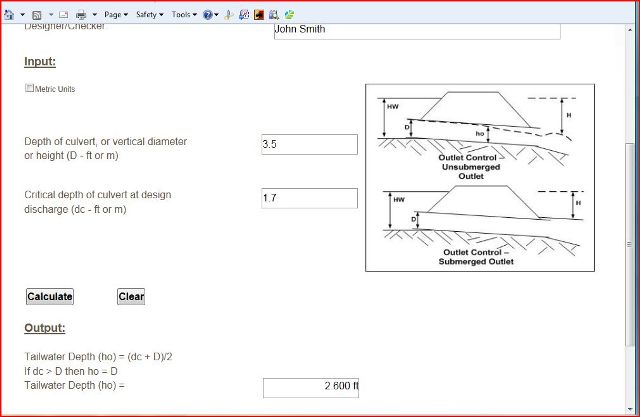11.
From the culverts submenu open up the headwater outlet control culvert calculation, CulvertHWOC.aspx. Input the "Title" of the calculation, the "Designer/Checker" information and the variables. Click on the "Calculate" button and read the results. The headwater depth for a round pipe culvert under outlet control is 2.442 ft.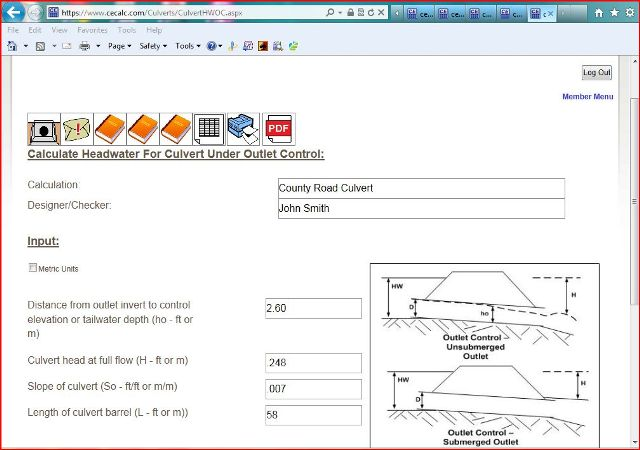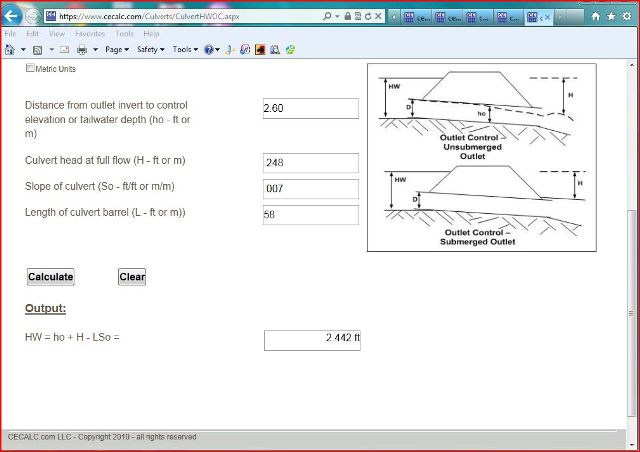12.
From the culverts submenu open up the culvert outlet velocity calculation, CulvertVout.aspx. Input the "Title" of the calculation, the "Designer/Checker" information and the variables. Click on the "Calculate" button and read the results. The outlet velocity is 3.638 fps.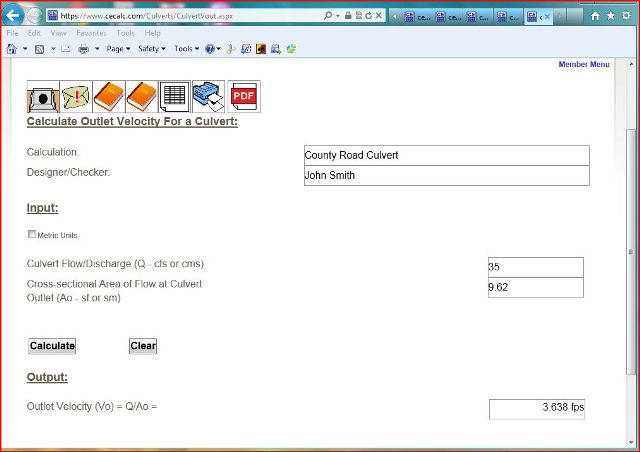13. Summary
Use a 42 inch concrete culvert pipe. Assume the culvert pipe is under inlet control with a headwater depth of 2.835 ft. Since the outlet velocity is 3.638 fps which is greater than scour velocity of 2 fps, provide erosion protection at the outlet for a minimum distance into the stream channel to prevent erosion.

 Start Using The Calculations >>> Become A Member Now!